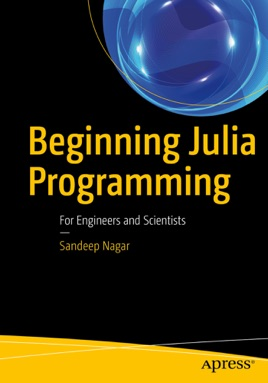• \$34.99

## Publisher Description

Get started with Julia for engineering and numerical computing, especially data science, machine learning, and scientific computing applications. This book explains how Julia provides the functionality, ease-of-use and intuitive syntax of R, Python, MATLAB, SAS, or Stata combined with the speed, capacity, and performance of C, C++, or Java. You’ll learn the OOP principles required to get you started, then how to do basic mathematics with Julia. Other core functionality of Julia that you’ll cover, includes working with complex numbers, rational and irrational numbers, rings, and fields.
Beginning Julia Programming takes you beyond these basics to harness Julia’s powerful features for mathematical functions in Julia, arrays for matrix operations, plotting, and more. Along the way, you also learn how to manage strings, write functions, work with control flows, and carry out I/O to implement and leverage the mathematics needed for your data science and analysis projects.
"Julia walks like Python and runs like C". This phrase explains why Julia is quickly growing as the most favored option for data analytics and numerical computation. After reading and using this book, you'll have the essential knowledge and skills to build your first Julia-based application.
You will:Obtain core skills in Julia
Apply Julia in engineering and science applications
Work with mathematical functions in Julia
Use arrays, strings, functions, control flow, and I/O in Julia
Carry out plotting and display basic graphics